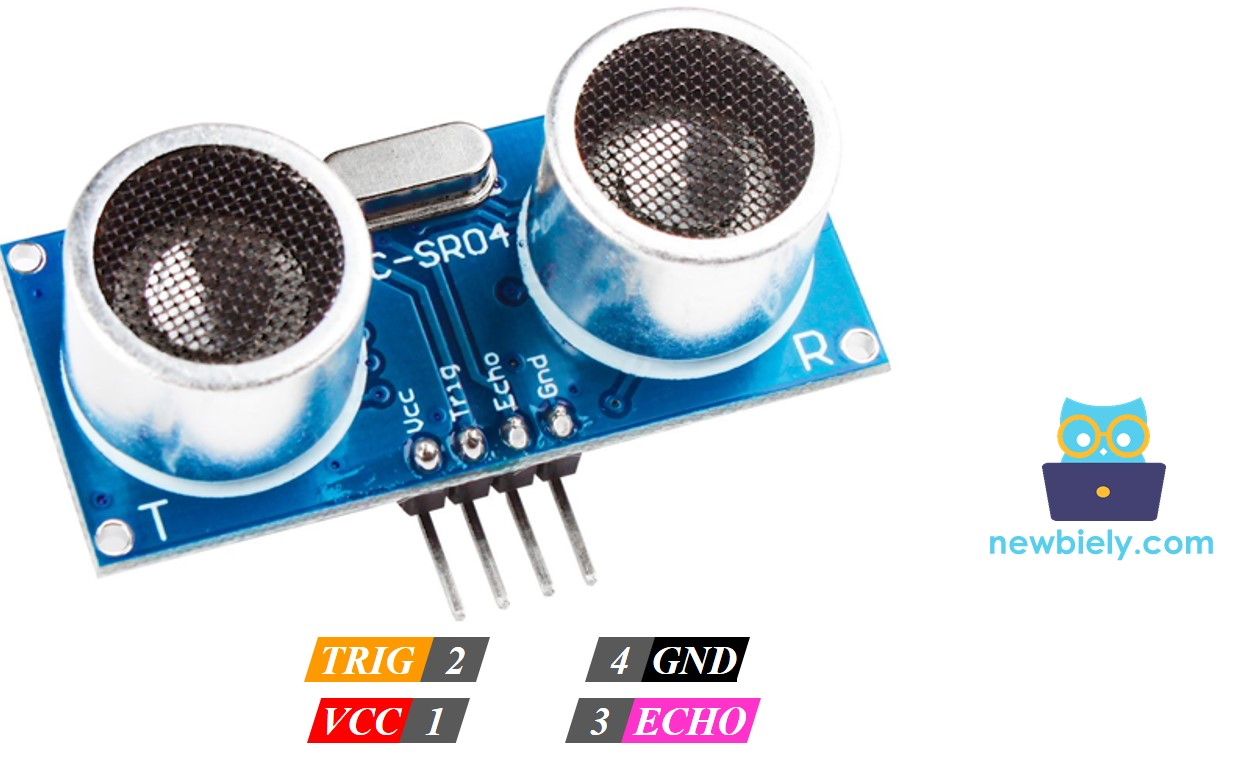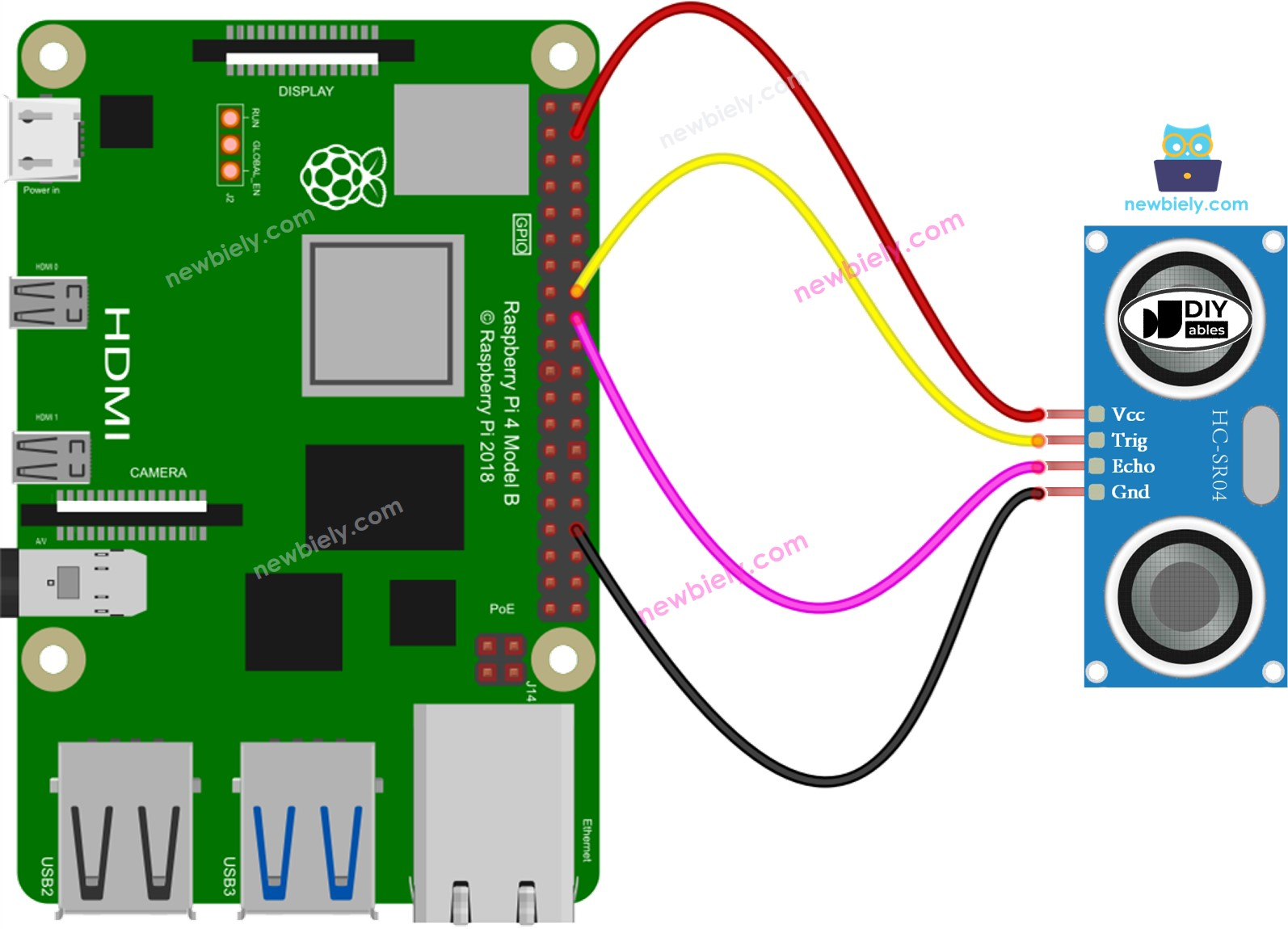# Raspberry Pi - Ultrasonic Sensor

This tutorial instructs you how to use Raspberry Pi and ultrasonic sensor to measure the distance to obstacles or objects. In detail, we will learn:

• How ultrasonic sensor works
• How to connect the ultrasonic sensor to Raspberry Pi
• How to program Raspberry Pi to measure distance using the ultrasonic sensor
• How to filter out noise from the distance measurements of the ultrasonic sensor in Raspberry Pi code

## Hardware Preparation

Or you can buy the following sensor kit:

## Overview of Ultrasonic Sensor

The HC-SR04 ultrasonic sensor is used to calculate the distance to an object by utilizing ultrasonic waves.

### The Ultrasonic Sensor Pinout

The HC-SR04 ultrasonic sensor has four pins:

• VCC pin: must be connected to VCC (5V)
• GND pin: must be connected to GND (0V)
• TRIG pin: this pin receives a control signal (pulse) from Raspberry Pi
• ECHO pin: this pin sends a signal (pulse) to Raspberry Pi. Raspberry Pi measures the length of the pulse to calculate the distance.### How It Works

This section includes advanced information that may be overwhelming. If you are unsure about the content, feel free to skip it and move on to the next sections.
1. The micro-controller generates a 10-microsecond pulse on the TRIG pin, which causes the ultrasonic sensor to automatically emit ultrasonic waves.
2. When the waves hit an obstacle, they are reflected back to the sensor.
3. The ultrasonic sensor then detects the reflected wave and measures its travel time.
4. As a result, the ultrasonic sensor generates a pulse to the ECHO pin, with the duration of the pulse being equal to the travel time of the ultrasonic wave.
5. The micro-controller measures the pulse duration in the ECHO pin and calculates the distance between the sensor and the obstacle.

### How to Get Distance From Ultrasonic Sensor

1. To calculate the distance from the ultrasonic sensor, two steps must be taken as outlined in the "How It Works" section (steps 1 and 6):
2. Generate a 10-microsecond pulse on the TRIG pin.
3. Measure the pulse duration in the ECHO pin.
4. Utilize the measured pulse duration to calculate the distance between the sensor and the obstacle.

#### Distance Calculation

We have:

• The travel time of the ultrasonic wave (µs): travel_time = pulse_duration
• The speed of the ultrasonic wave: speed = SPEED_OF_SOUND = 340 m/s = 0.034 cm/µs

So:

• The travel distance of the ultrasonic wave (cm): travel_distance = speed × travel_time = 0.034 × pulse_duration
• The distance between sensor and obstacle (cm): distance = travel_distance / 2 = 0.034 × pulse_duration / 2 = 0.017 × pulse_duration

### Raspberry Pi - Ultrasonic Sensor

The pins of a Raspberry Pi can create a 10-microsecond pulse and measure the length of the pulse. This allows us to calculate the distance from the ultrasonic sensor by using two Raspberry Pi pins. So, we just need to use two Raspberry Pi's pins:

• One pin is connected to the TRIG pin of the ultrasonic sensor in order to generate a 10µs pulse.
• The other pin is connected to the ECHO pin of the ultrasonic sensor to measure the pulse from the sensor

## Wiring DiagramThis image is created using Fritzing. Click to enlarge image

## How To Program For Ultrasonic Sensor

• Create a 10-microsecond pulse on Raspberry Pi's pin 9 by utilizing the GPIO.output() and time.sleep() functions.
• For instance:
• Set pin 9 to HIGH with digitalWrite() and then delay for 10 microseconds with delayMicroseconds().
GPIO.output(TRIG_PIN, GPIO.HIGH) time.sleep(0.00001) GPIO.output(TRIG_PIN, GPIO.LOW)
• Determine the pulse duration (µs) on Raspberry Pi's pin 8 by using the pulseIn() function. For example:
while GPIO.input(ECHO_PIN) == 0: pulse_start = time.time() while GPIO.input(ECHO_PIN) == 1: pulse_end = time.time() pulse_duration = pulse_end - pulse_start
• Determine the distance (cm):
distance = pulse_duration * 34300 / 2

## Raspberry Pi Code

### Detailed Instructions

• Make sure you have Raspbian or any other Raspberry Pi compatible operating system installed on your Pi.
• Make sure your Raspberry Pi is connected to the same local network as your PC.
• Make sure your Raspberry Pi is connected to the internet if you need to install some libraries.
• If this is the first time you use Raspberry Pi, See how to set up the Raspberry Pi
• Connect your PC to the Raspberry Pi via SSH using the built-in SSH client on Linux and macOS or PuTTY on Windows. See to how connect your PC to Raspberry Pi via SSH.
• Make sure you have the RPi.GPIO library installed. If not, install it using the following command:
sudo apt-get update sudo apt-get install python3-rpi.gpio
• Create a Python script file ultrasonic_sensor.py and add the following code:
# This Raspberry Pi code was developed by newbiely.com # This Raspberry Pi code is made available for public use without any restriction # For comprehensive instructions and wiring diagrams, please visit: # https://newbiely.com/tutorials/raspberry-pi/raspberry-pi-ultrasonic-sensor import RPi.GPIO as GPIO import time # Set the GPIO mode (BCM or BOARD) GPIO.setmode(GPIO.BCM) # Define GPIO pins for the ultrasonic sensor TRIG_PIN = 23 ECHO_PIN = 24 # Set the trigger and echo pins GPIO.setup(TRIG_PIN, GPIO.OUT) GPIO.setup(ECHO_PIN, GPIO.IN) def get_distance(): # Send a short pulse to the trigger pin GPIO.output(TRIG_PIN, GPIO.HIGH) time.sleep(0.00001) GPIO.output(TRIG_PIN, GPIO.LOW) # Measure the duration for the echo pulse while GPIO.input(ECHO_PIN) == 0: pulse_start = time.time() while GPIO.input(ECHO_PIN) == 1: pulse_end = time.time() pulse_duration = pulse_end - pulse_start # Calculate the distance based on the speed of sound (34300 cm/s) distance = pulse_duration * 34300 / 2 return distance try: while True: distance = get_distance() print(f"Distance: {distance:.2f} cm") time.sleep(1) except KeyboardInterrupt: # If the user presses Ctrl+C, clean up the GPIO configuration GPIO.cleanup()
• Save the file and run the Python script by executing the following command in the terminal:
python3 ultrasonic_sensor.py
• Place your hand in front of the ultrasonic sensor
• Observe the distance between the sensor and your hand displayed on the Terminal
PuTTY - Raspberry Pi
distance: 29.4 cm distance: 27.6 cm distance: 26.9 cm distance: 17.4 cm distance: 16.9 cm distance: 14.3 cm distance: 15.6 cm distance: 13.1 cm

The script runs in an infinite loop continuously until you press Ctrl + C in the terminal.

### Code Explanation

Check out the line-by-line explanation contained in the comments of the source code!

## How to Filter Noise from Distance Measurements of Ultrasonic Sensor

The measurement result from an ultrasonic sensor may contain noise which can lead to undesired operation in some applications. To remove this noise, the following algorithm can be used:

1. Take multiple measurements and store them in an array
2. Sort the array in ascending order
3. Filter out the noise by:
• The smallest samples are considered as noise and should be ignored
• The biggest samples are considered as noise and should be ignored
• The average of the middle samples should be taken as measured value

The following example code takes 20 measurements:

• Ignore the five smallest samples, which are considered as noise.
• Ignore the five biggest samples, which are also considered as noise.
• Get the average of the 10 middle samples (from 5th to 14th).
# This Raspberry Pi code was developed by newbiely.com # This Raspberry Pi code is made available for public use without any restriction # For comprehensive instructions and wiring diagrams, please visit: # https://newbiely.com/tutorials/raspberry-pi/raspberry-pi-ultrasonic-sensor import RPi.GPIO as GPIO import time TRIG_PIN = 23 # Raspberry Pi GPIO pin connected to TRIG pin of ultrasonic sensor ECHO_PIN = 24 # Raspberry Pi GPIO pin connected to ECHO pin of ultrasonic sensor GPIO.setmode(GPIO.BCM) GPIO.setup(TRIG_PIN, GPIO.OUT) GPIO.setup(ECHO_PIN, GPIO.IN) def get_distance(): filter_array = [] num_samples = 20 # Taking multiple measurements and store in an array for _ in range(num_samples): filter_array.append(ultrasonic_measure()) time.sleep(0.03) # To avoid ultrasonic interference (30 milliseconds delay) # Sorting the array in ascending order filter_array.sort() # Filtering noise # Discard the five smallest and five largest samples filtered_samples = filter_array[5:-5] # Calculate the average of the remaining samples distance = sum(filtered_samples) / len(filtered_samples) return distance def ultrasonic_measure(): GPIO.output(TRIG_PIN, True) time.sleep(0.00001) GPIO.output(TRIG_PIN, False) while GPIO.input(ECHO_PIN) == 0: pulse_start = time.time() while GPIO.input(ECHO_PIN) == 1: pulse_end = time.time() pulse_duration = pulse_end - pulse_start distance_cm = 0.017 * pulse_duration return distance_cm try: while True: distance = get_distance() print("Distance: {:.2f} cm".format(distance)) time.sleep(1) # Wait for 1 second before taking the next measurement except KeyboardInterrupt: print("Measurement stopped by the user.") finally: GPIO.cleanup()

## Video Tutorial

Creating videos is a time-consuming task. If you find video tutorials helpful for your learning, please let us know by subscribing to our YouTube channel . If there is high demand for videos, we will work on creating them.

## Challenge Yourself

Utilize an ultrasonic sensor to complete one of the following projects:

• Construct a collision avoidance system for a remote-controlled car.
• Measure the amount of material in a dustbin.
• Monitor the level of the dustbin.
• Automatically open and close the dustbin. Hint: Refer to Raspberry Pi - Servo Motor.

## Ultrasonic Sensor Applications

• Avoiding Collisions
• Detecting Fullness
• Measuring Level
• Detecting Proximity